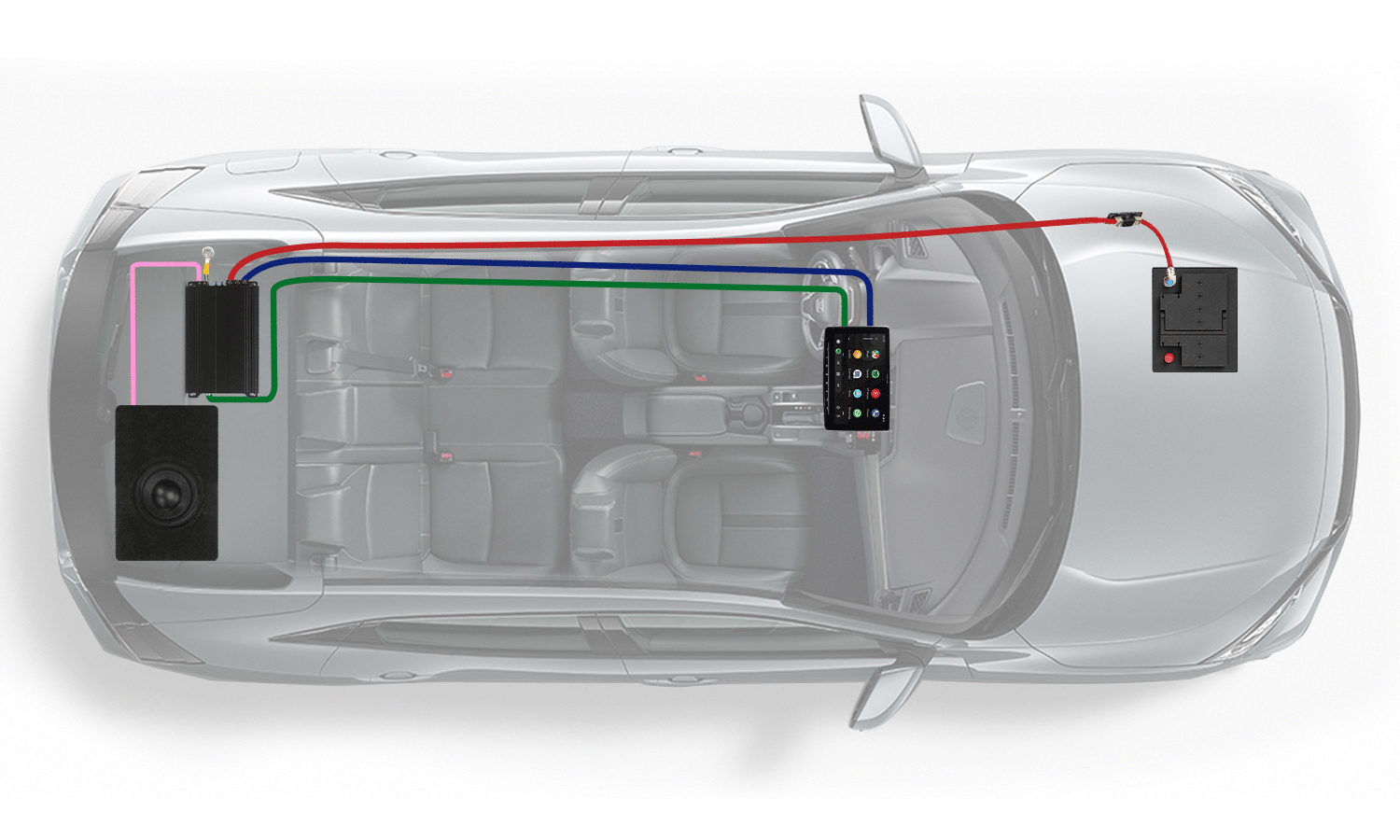### HOW TO WIRE SUBS

##### The secret to make your bass sound great!

The secret to great bass is making sure your subwoofers and amp are evenly matched and will properly work together. And this article will help you figure out how to do just that — match amplifiers and subwoofers.

Getting the right sub for an amp, or the right amp for a sub, is like solving a puzzle. A subwoofer has impedance (measured in ohms) which represents the "load" an amplifier will sense on its output. An amplifier will put out different amounts of power (watts RMS) based on the impedance load it "sees."

##### Solving the puzzle

The amplifier's capabilities (X watts RMS into Y ohms) needs to match the subwoofer's specifications (Y ohms and can handle X watts HOW TO WIRE SUBS RMS). Your goal is to get those two variables to match for both the amplifier and the subwoofer. Below, we cover the important basics of powermatching, impedance, and planning for the number of subs you want, and we approach the situation from both sides of the system:

• Part A: You have the subs, which amp should you get?
• Part B: You have the amp, which subs should you get?
• And also, Part C: You have neither amp nor sub, and want help deciding how to begin. Start with either part you want, but A and B are both worth a read.

##### Part A — You have the subs, which amplifier should you get?

The subwoofers need to be the same Multiple subs wired together must be the same coil type and impedance. If they’re not, the power won’t divide evenly between them, and some subs would probably be over-powered while others get underpowered. If you want to run different types of subs in a system, each type needs to have its own separate amp.

Step 1: How much power? Find out the “watts RMS” rating of the sub Then, multiply the number of subs you have by the RMS rating of each, to get their total RMS rating. You want to make sure the amp you choose will supply no more than the sub system’s total RMS rating.

Step 2: What impedance? The results of combining coils and subs Figure out the possible total impedance(s) that the subs can be wired together to form

(SVC = single voice coil, 1 pair of terminals; DVC = dual voice coil, 2 pairs of terminals.)

For more combinations of subs and their impedances, see Subwoofer Wiring Diagrams.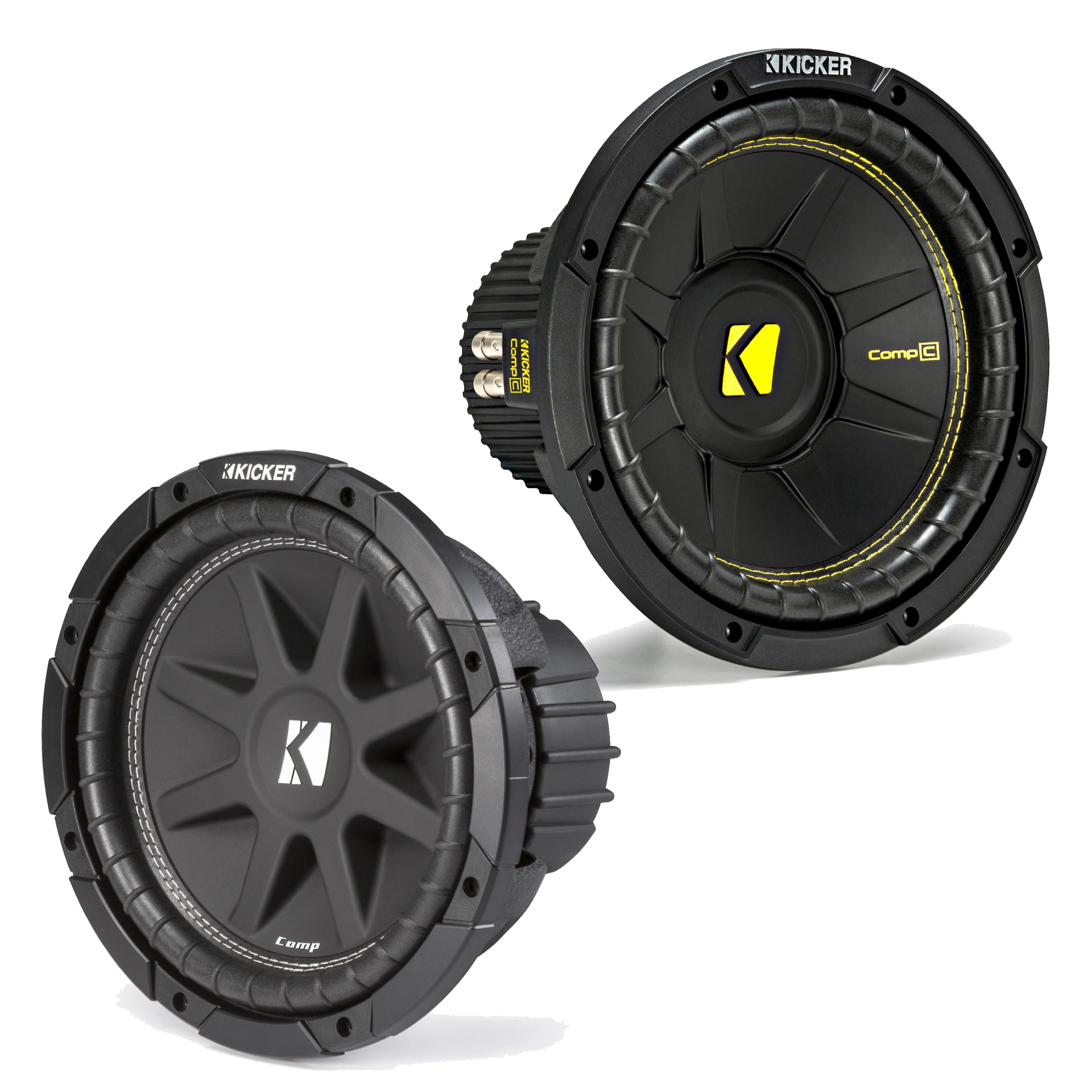Step 3: Pick an amp that can do both — X watts at Y ohms

Look for an amplifier that can put out power up to the RMS wattage you’ve figured in Step 1, at an impedance load the subs can be wired to form, from Step 2 Estimating amp power at the odd impedance values:
• 8 ohms — figure on the amp putting out half the power it would at 4 ohms
• 6 ohms — figure on the amp putting out threequarters of the power it would at 4 ohms
• 3 ohms — figure on the amp putting out the average of what it would at 2 ohms and at 4 ohms
• 2.7 ohms — figure the same as for 3 ohms, and add a few watts
• 1.3 ohms — use the 1-ohm spec and take away a few watts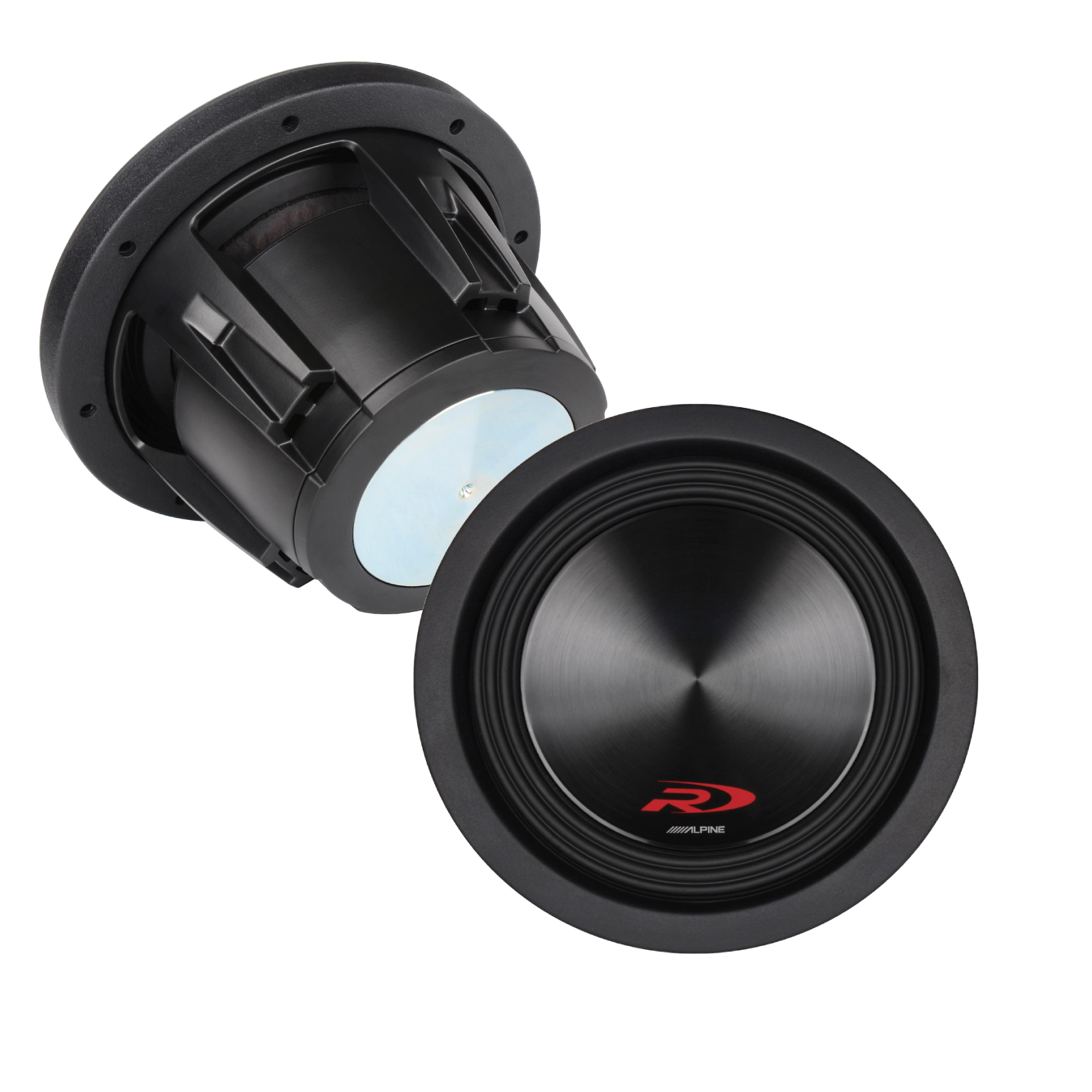Example: You have two Alpine Type R SWR-8D4 8". subwoofers and you want the right amp for them.

They are DVC 4-ohm subs rated at 350 watts RMS each. Two 350 watts RMS subs together need a total of 700 watts RMS. Using the chart in Step 2, 2 DVC 4-ohm subs can be wired together to form a 1-ohm, a 4-ohm, or a 16- ohm load.

The last is too high a load to be practical, so you’ll look for an amp that can put out up to 700 watts RMS into either a 4-ohm load, or a 1-ohm impedance load:

• • up to 700 watts RMS x 1 at 4 ohms, or
• • up to 700 watts RMS x 1 at 1 ohm
•
Among Online Car Stereo selection of amplifiers you’ll find:
Any one of these high-quality amplifiers would work well with those subs. It doesn’t matter which impedance an amp plays through — 600 watts RMS through a 4-ohm load produces the same volume as 600 watts RMS through a 1-ohm load.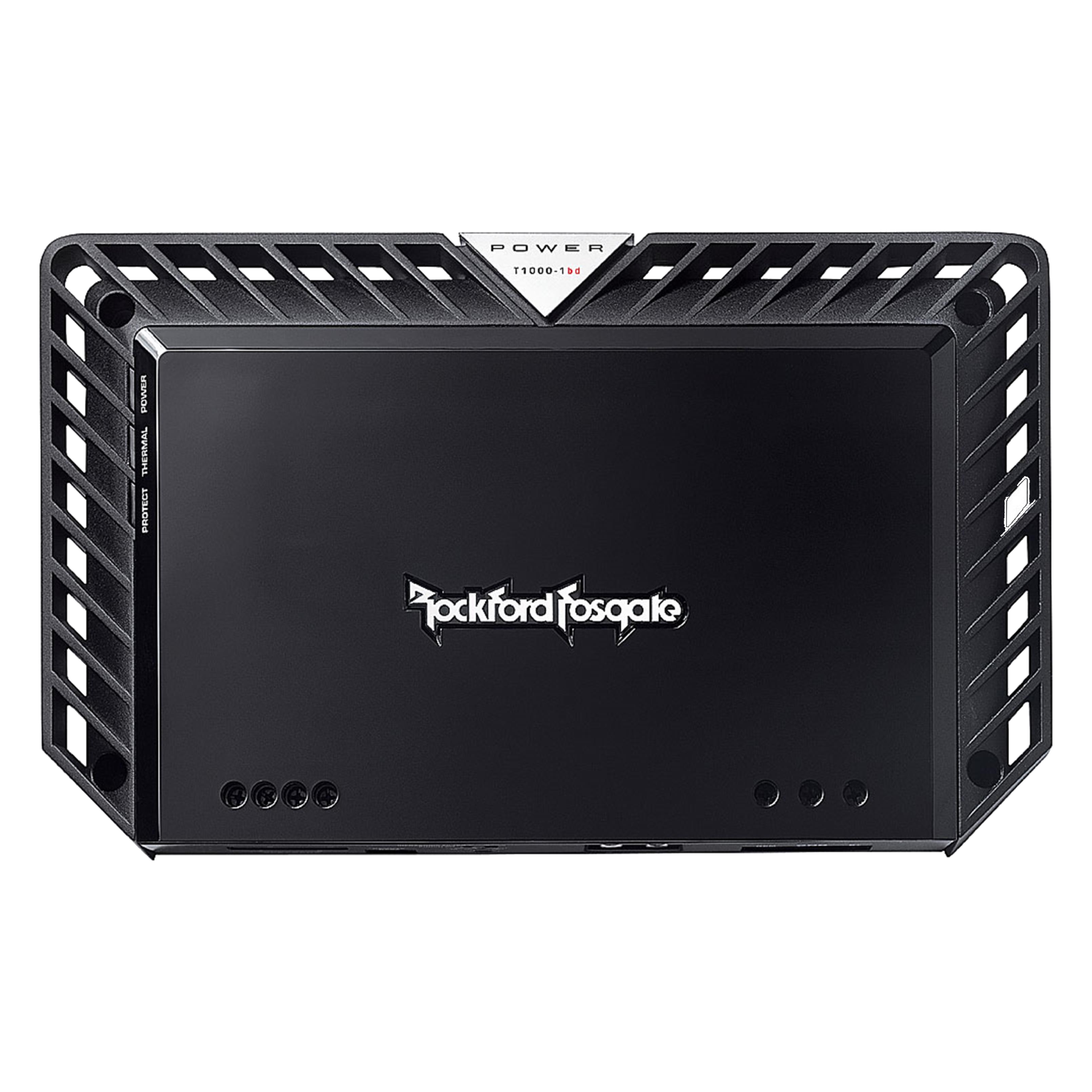##### Part B — You have the amplfier, which subs should you get?

Step 1: What can the amp do? Find the RMS ratings of the amp at different loads Find the amp’s power, expressed in “watts RMS”, at 4 ohms, at 2 ohms, and, if it can, at 1 ohm. Pick the power you’d like to achieve. The load impedance (ohms) of that rating will be what you want your subs’ total impedance to be.

Step 2: How many subs do you want? Divide the power you picked in Step 1 by the number of subs you want. This number is the target RMS rating for each of the subs you’ll choose. Step 3: What impedance does each sub need to be and how many voice coils? Using the impedance you picked in Step 1 and the number of subs from Step 2, cross-reference the possible coil configurations that you can use:

# Subwoofers1-ohm2-ohms4-ohms
1 SubwooferDVC 2-ohmsSVC 2-ohms
DVC 4-ohms
SVC 4-ohms
DVC 2-ohms
2 SubwooferSVC 2-ohms
DVC 4-ohms
SVC 4-ohms
DVC 2-ohms
SVC 2-ohms
DVC 4-ohms
3 Subwoofer (1.3 ohms)*
SVC 4-ohms
DVC 2-ohms
(3 or 2.7 ohms)*
DVC 2-ohms
DVC 4-ohms
(6 ohms)*
SVC 2-ohms
DVC 4-ohms
4 SubwooferSVC 4-ohms
DVC 2-ohms
SVC 2-ohms
DVC 4-ohms
SVC 4-ohms
DVC 2-ohms
* Estimate amp power at the odd impedance values like in Part A, Step 3, above. Step 4: Pick a sub that works for both — (SVC or DVC) X-ohms, Y watts RMS) Look for subs that are rated at the wattage you figured in Step 2, and are configured as you found in Step 3. This might sound confusing, so let's walk through an example and it'll make sense.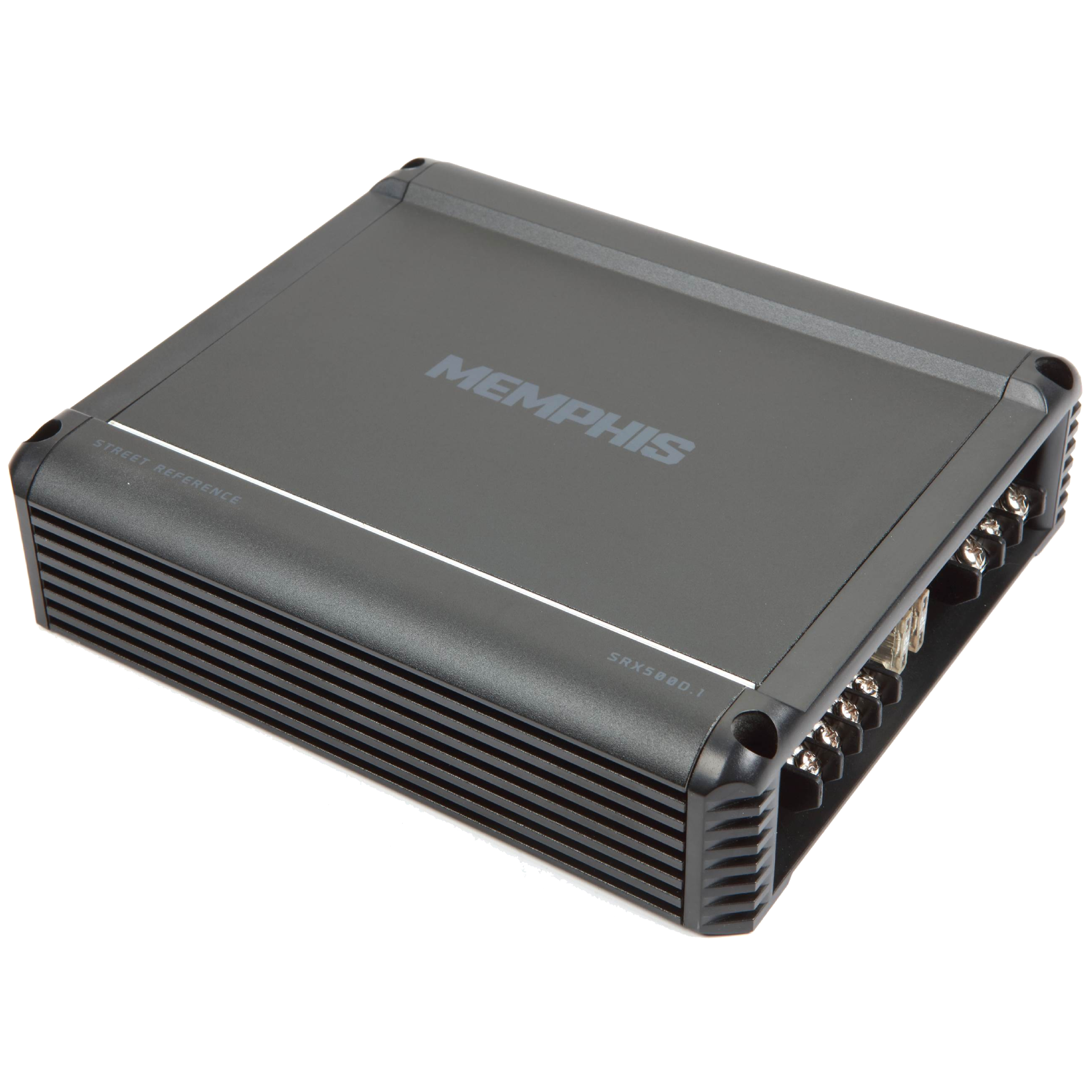Example: You have a Memphis Audio SRX500D.1 amplifier and you want it to drive two subwoofers The amp is capable of 350 watts RMS x 1 at 4 ohms and 500 watts RMS x 1 at 2 ohms. Let’s say you choose to maximize the amp’s potential and want the system to put out 500 watts RMS. This means your subs have to be wired to form a total impedance of 2 ohms. Two subs on a 500 watts RMS amp will want about 250 watts RMS each. So you’ll look for subs each rated for 250 watts RMS or more. Using the chart in Step 3, for two subwoofers, a final 2-ohm load can be achieved with either two SVC 4- ohm subs or two DVC 2-ohm subs. So, you’ll look for two subs that are either SVC 4- ohms or DVC 2-ohms, rated for at least 250 watts RMS each:
•
• • 2 SVC 4-ohms, at least 250 watts RMS, or
• • 2 DVC 2-ohms, at least 250 watts RMS
•
Among OnlineCarStereo’s selection of subwoofers you’ll find:

Kicker CompC 10" component subwoofer All these subwoofers will sound their best when amplified with the proper amount of power. Differences in size have more to do with tonal qualities and frequency response than with power performance. And optimizing performance is the point of matching subs and amps together.

##### Part C — You have neither amp nor sub, and want help deciding how to begin### (Click on the components to see instructions.)